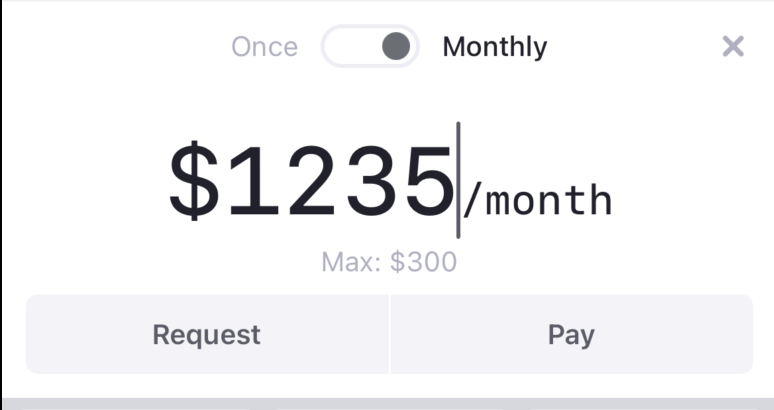Issue #845

Suppose we have struct `Payment` as the state, we declare custom `Binding<String>` that derives from our state.

``````struct Payment {
var amount: Int = 0
}
``````

To show our suffix view, we use `.fixedSize(horizontal: true, vertical: false)` to constrain our `TextField` to fit content, and to avoid it to grow outside our frame, we constrain a max limit, like `10_000` in this case. An interesting thing is we divide by 10 to not let the newly typed digit enter if the amount is above our limit

Note also the use of `firstTextBaseline` in `HStack` to align baseline``````var textField: some View {
let text = Binding<String>(
get: {
state.payment.amount > 0 ? "\$\(state.payment.amount)" : ""
},
set: { text in
let text = text.replacingOccurrences(of: "\$", with: "")
let amount = Int(text) ?? 0
if amount < 10_000 {
state.payment.amount = amount
} else {
state.payment.amount = amount / 10
}
}
)

return HStack(alignment: .firstTextBaseline, spacing: 0) {
TextField("\$0", text: text)
.fixedSize(horizontal: true, vertical: false)
.font(.system(size: 48, weight: .medium, design: .monospaced))
.foregroundColor(Asset.textPrimary.color)
.multilineTextAlignment(.center)
.accentColor(Asset.orange.color)

if state.payment.frequency == .monthly {
Text("/month")
.font(.system(size: 21, weight: .medium, design: .monospaced))
.foregroundColor(
state.payment.amount > 0 ? Asset.textPrimary.color : Asset.textSecondary.color
)
}
}
}

``````